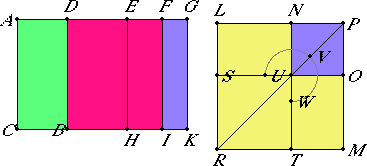# Proposition 91

If an area is contained by a rational straight line and a first apotome, then the side of the area is an apotome.

Let the area AB be contained by the rational straight line AC and the first apotome AD.

I say that the side of the area AB is an apotome.Since AD is a first apotome, let DG be its annex, therefore AG and GD are rational straight lines commensurable in square only. Also, the whole AG is commensurable with the rational straight line AC set out, and the square on AG is greater than the square on GD by the square on a straight line commensurable in length with AG.

X.17

Therefore if there is applied to AG a parallelogram equal to the fourth part of the square on DG and deficient by a square figure, then it divides it into commensurable parts.

Bisect DG at E, apply to AG a parallelogram equal to the square on EG and deficient by a square figure, and let it be the rectangle AF by FG. Then AF is commensurable with FG.

Draw EH, FI, and GK through the points E, F, and G parallel to AC.

X.15

Now, since AF is commensurable in length with FG, therefore AG is also commensurable in length with each of the straight lines AF and FG.

X.12

But AG is commensurable with AC, therefore each of the straight lines AF and FG is commensurable in length with AC.

X.19

And AC is rational, therefore each of the straight lines AF and FG is also rational, so that each of the rectangles AI and FK is also rational.

X.15

Now, since DE is commensurable in length with EG, therefore DG is also commensurable in length with each of the straight lines DE and EG.

But DG is rational and incommensurable in length with AC, therefore each of the straight lines DE and EG is also rational and incommensurable in length with AC. Therefore each of the rectangles DH and EK is medial.

VI.26

Now make the square LM equal to AI, and subtract the square NO having a common angle with it, the angle LPM, and equal to FK. Then the squares LM and NO are about the same diameter.

Let PR be their diameter, and draw the figure.

VI.17

Since the rectangle AF by FG equals the square on EG, therefore AF is to EG as EG is to FG.

But AF is to EG as AI is to EK, and EG is to FG as EK is to KF, therefore EK is a mean proportional between AI and KF.

X.54's Lemma

But it was proved before that MN is also a mean proportional between LM and NO, and AI equals the square LM, and KF equals NO, therefore MN also equals EK.

But EK equals DH, and MN equals LO, therefore DK equals the gnomon UVW and NO.

But AK also equals the sum of the squares LM and NO, therefore the remainder AB equals ST.

But ST is the square on LN, therefore the square on LN equals AB. Therefore LN is the side of AB.

I say next that LN is an apotome.

Since each of the rectangles AI and FK is rational, and they equal LM and NO, therefore each of the squares LM and NO, that is, the squares on LP and PN respectively, is also rational. Therefore each of the straight lines LP and PN is also rational.

Again, since DH is medial and equals LO, therefore LO is also medial.

Since, then, LO is medial, while NO is rational, therefore LO is incommensurable with NO.

But LO is to NO as LP is to PN, therefore LP is incommensurable in length with PN.

X.73

And both are rational, therefore LP and PN are rational straight lines commensurable in square only. Therefore LN is an apotome.

And it is the side of the area AB, therefore the side of the area AB is an apotome.

Therefore, if an area is contained by a rational straight line and a first apotome, then the side of the area is an apotome.

Q.E.D.

## Guide

This proposition is used in X.108.